Next: muon background Up: Collimation Previous: material

## wakefield

K.Yokoya derived a theoretical expression of wakefield induced by the collimators, which shall be compared to a measurement. The emittance growth due to the wakefield is expressed by,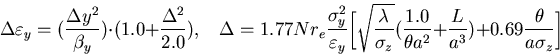(3)
where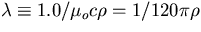is the resistive depth of material of the collimator, and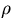is the conductivity, and N,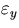,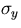,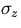are the intensity/bunch, (vertical) invariant emittance, vertical beam size, bunch length of the beam, respectively. re is the electron classical radius. The geometrical parameters of the collimator are displayed in Fig.3.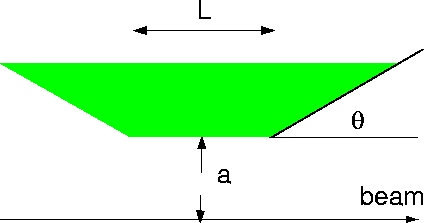There is the optimal angle (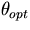) minimizing the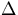, that is,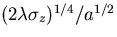. With this angle and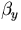instead ofand, theis,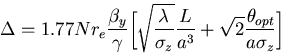(4)
where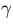is the Lorentz factor, Ebeam /me.

The emittance growth should be less than 1% so that the total growth due to 6 collimators is less than 6%. Assuming that the surface of the collimator is plated by copper, the aperture (a) of the collimator must be 250 (204)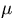m corresponding to 70.4 (56.9)with the length of 20 (10)cm, N = 1010/bunch=80m and=400m at Ebeam=750GeV, where the optimal angle () is 1.05o (1.17o) .Next: muon background Up: Collimation Previous: material
Toshiaki Tauchi
12/20/1999